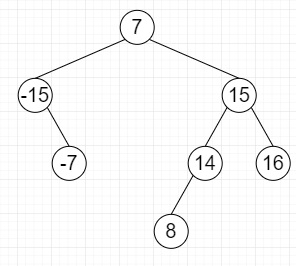# Find if there is a triplet in a Balanced BST that adds to zero in C++

Suppose we have a balanced binary search tree, we have to create a function named is_valid_triplet() that returns true when there exist a triplet in given BST whose sum equals to 0, otherwise returns false. Design the method by following these constraints −

• expected time complexity is O(n^2)

• O(logn) extra space can be used.

So, if the input is likethen the output will be True, as triplet is [-15,7,8]

To solve this, we will follow these steps −

• Define a function bst_to_doubli_list(), this will take root, head, tail,

• if root is same as NULL, then −

• return

• if left of root is not null, then −

• bst_to_doubli_list(left of root, head, tail)

• left of root := tail

• if tail is not null , then −

• right of tail := root

• Otherwise

• tail := root

• if right of root is not null, then −

• bst_to_doubli_list(right of root, head, tail)

• Define a function is_in_double_list(), this will take head, tail, sum,

• while head is not equal to tail, do −

• current := key of head + key of tail

• if current is same as sum, then −

• return true

• otherwise when current > sum, then −

• tail := left of tail

• Otherwise

• return false

• From the main method, do the following −

• if root is null, then −

• return false

• tail = null

• while (right of head is not equal to tail and key of head < 0), do −

• if is_in_double(right of head, tail, key of head * (-1), then

• return true

• Otherwise

• return false

## Example (C++)

Let us see the following implementation to get better understanding −

Live Demo

#include <bits/stdc++.h>
using namespace std;
class TreeNode {
public:
int key;
TreeNode *left;
TreeNode *right;
TreeNode() : key(0), left(NULL), right(NULL) {}
TreeNode(int x) : key(x), left(NULL), right(NULL) {}
};
void bst_to_doubli_list(TreeNode* root, TreeNode** head, TreeNode** tail) {
if (root == NULL)
return;
if (root->left)
root->left = *tail;
if (*tail)
(*tail)->right = root;
else
*tail = root;
if (root->right)
}
bool is_in_double_list(TreeNode* head, TreeNode* tail, int sum) {
int current = head->key + tail->key;
if (current == sum)
return true;
else if (current > sum)
tail = tail->left;
else
}
return false;
}
bool is_valid_triplet(TreeNode *root) {
if (root == NULL)
return false;
TreeNode* tail = NULL;
return true;
else
}
return false;
}
TreeNode* insert(TreeNode* root, int key) {
if (root == NULL)
return new TreeNode(key);
if (root->key > key)
root->left = insert(root->left, key);
else
root->right = insert(root->right, key);
return root;
}
int main(){
TreeNode* root = NULL;
root = insert(root, 7);
root = insert(root, -15);
root = insert(root, 15);
root = insert(root, -7);
root = insert(root, 14);
root = insert(root, 16);
root = insert(root, 8);
cout << is_valid_triplet(root);
}

## Input

root = insert(root, 7);
root = insert(root, -15);
root = insert(root, 15);
root = insert(root, -7);
root = insert(root, 14);
root = insert(root, 16);
root = insert(root, 8);

## Output

1